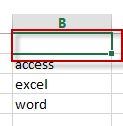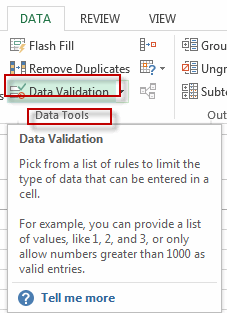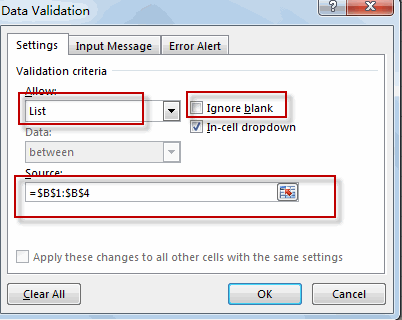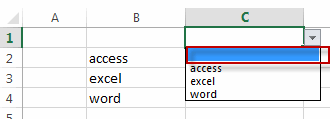Add Blank in Data Validation List

This post will guide how to add blank as one option in the data validation list in excel. How do I add a blank in data validation list. How to add a data validation drop-down list with empty cell as an option in excel. How to put a blank option into a data validation list as the first option of the drop-down list in excel.

Add Blank in Data Validation List

If you have a list of data in your worksheet and you have created one data validation drop-down list and you want to put a blank into the existing data validation list as the first one option in the drop-down list. You can do the following steps:

#1 you need to add one blank cell above the data list that be used to create a data validation list.#2 select one blank cell that you want to add the data validation list. Such as: Cell C1

#3 go to DATA tab, click Data Validation command under Data Tools group. The Data Validation dialog will appear.#4 select List option form the drop-down list of Allow list box, and uncheck the checkbox of Ignore blank, then select the source cell.#5 click OK button.You will see that a blank option has been added into the data validation list.

Related Posts

Extract Unique Items From A List

This post will guide you how to extract unique itmes from a given list in Microsoft Excel. How to create a newly formula to get unique values from a range cells in Excel. The unique list of items is the ...

Extract substring In Excel

This post will guide you how to use Excel's MID function is a quick and easy way to extract pieces from your text. Use the Excel formula to extract a substring with MID. Note: If you want to extract just ...

How to Use 3D SUM Multiple Worksheets

To sum a range of numbers is straightforward for most Excel users, but do you know how to establish a 3D reference to total the same range of numerous sheets. In this post, I will present the steps for this ...

Extract all the matches with helper Column

With Excel's powerful functions IF, INDEX, and MATCH, we can find exactly what you're looking for with a few clicks of the mouse. This step-by-step tutorial will show how easy it is to extract data using these tools and more! ...

If Cell is This Value or That Value

IF function is frequently used in Excel worksheet to return you expect “true value” or “false value” based on the result of logical test. If you want to see if a cell is A or B, and if one of ...

If Value is Greater Than A Certain Value

IF function is frequently used in Excel worksheet to return you expect “true value” or “false value” based on the logical test result. If you want to see if a value in one cell is greater than a specific value, ...

If Cell is Not Blank

IF function is frequently used in Excel worksheet to return you expect “true value” or “false value” based on the result of created logical test. If you want to see if a cell is blank or not, and leave some ...

VBA Macro For VLOOKUP From Another Sheet

In the previous post, you should know that how to fix or remove the #N/A error when using VLOOKUP formula to lookup value from another sheet. And this post will show you how to use VBA code to vlookup data ...

If Cell is Blank

IF function is frequently used in Excel worksheet to return you expect “true value” or “false value” based on the result of created logical test. If you want to see if a cell is blank or not, and leave some ...

If Cell Equals Certain Text String

IF function is frequently used in Excel worksheet to return you expect “true value” or “false value” based on the result of created logical test. If you want to see if cell equals a certain text string like “Win”, you ...

Sidebar